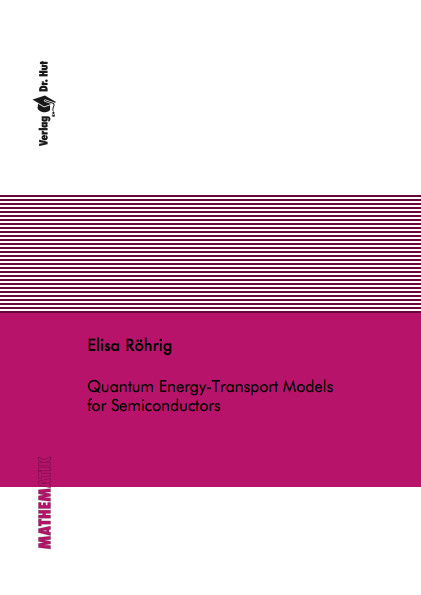Datenbestand vom 25. Mai 2020aktualisiert am 25. Mai 2020

# ISBN 9783843904957Euro 60,00 inkl. 7% MwSt

978-3-8439-0495-7, Reihe Mathematik

Elisa Röhrig
Quantum Energy-Transport Models for Semiconductors

101 Seiten, Dissertation Technische Universität Kaiserslautern (2012), Softcover, A5

## Zusammenfassung / Abstract

We consider energy-transport (ET) models and quantum energy-transport (QET) models for semiconductors. Quantum energy-transport models contain a quantum correction, which plays an important role as soon as the considered devices are of small size. Both macroscopic models offer a good balance of physical precision and cost for numerical computation, in particular if temperature effects play a role. They can be derived in the zero relaxation time limit from the hydrodynamic and the quantum hydrodynamic model, respectively. Additionally to the electron density the electron temperature is an unknown in these models. ET models were used in the past for simulations, for example for modeling heating in semiconductor devices. The question whether energy-transport models have unique weak solutions has not been answered yet. We determine here the analytical properties of energy-transport and quantum energy-transport models. In the first part we derive a bipolar energy-transport model for a MOS diode and provide an existence analysis. In the second part we show existence of a weak solution to a transient energy-transport model, which is unique, if a higher regularity is assumed. In the third part we consider a stationary quantum energy-transport model. For temperatures close to constant temperature we show existence of weak solutions for arbitrarily large applied voltages. These solutions are unique if the applied voltage is small. The proof is based on a decoupling strategy, which also gives a robust method for the numerical computation of the solution to the equations. In the last part we investigate the stability properties of stationary states in the corresponding transient QET model.